Courses

# Nine members of a family are standing in a row. Their average age is 70. The average age of the first four members in the row is 64 and the average age of the last seven members in the row is 80. What is the average age of the third and fourth member in the row?a)78b)86c) 93d) 89e)87Correct answer is option 'C'. Can you explain this answer? Related Test: NMAT Quantitative Skills MCQ Quiz - 3

## CAT Question

By Ranjeet · Dec 27, 2019 ·CAT
Shruti Jain answered Dec 27, 2019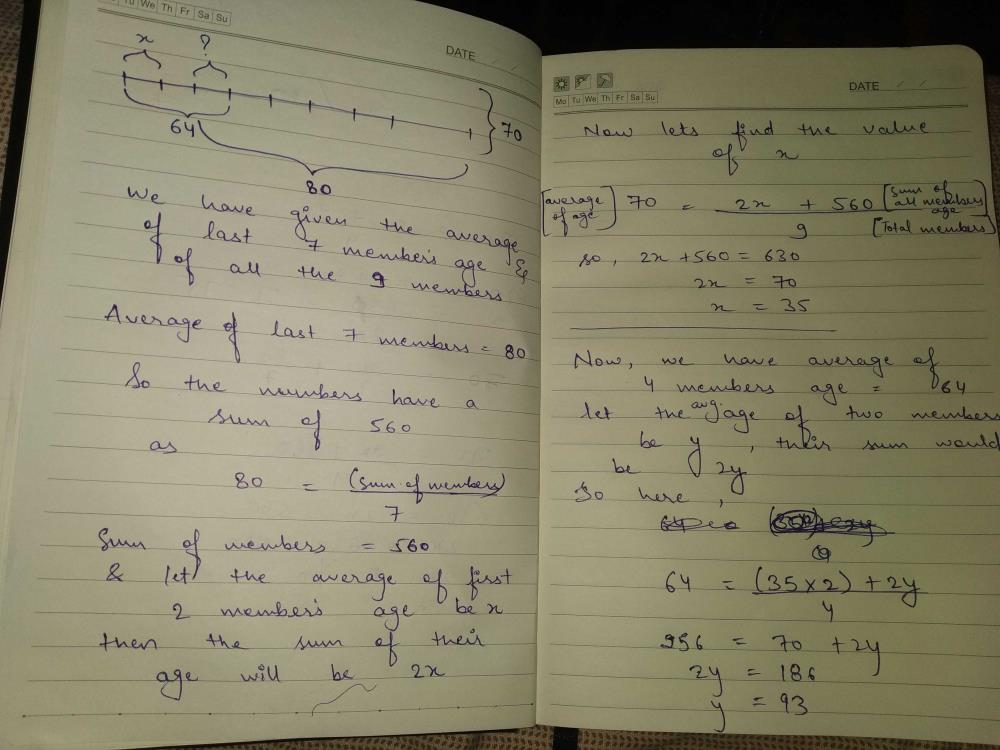Venky Dhawan answered Apr 21, 2020
The avg. age of 9 members is 70
so s1+ s2 + to s9/ 9= 70 = 70×9 = 630
as the avg. age of last 7 member is 80

80× 7 = 560 now..
630 - 560= 70
which is the combined age of s1 and s2.
as it is giver avg of first 4 members is 64 so the combined age is 256

from which 256 - 70= 186

which is combined age of s3 and s4 so the avg is 186/ 2 = 93.

Gurvani Monga answered May 07, 2020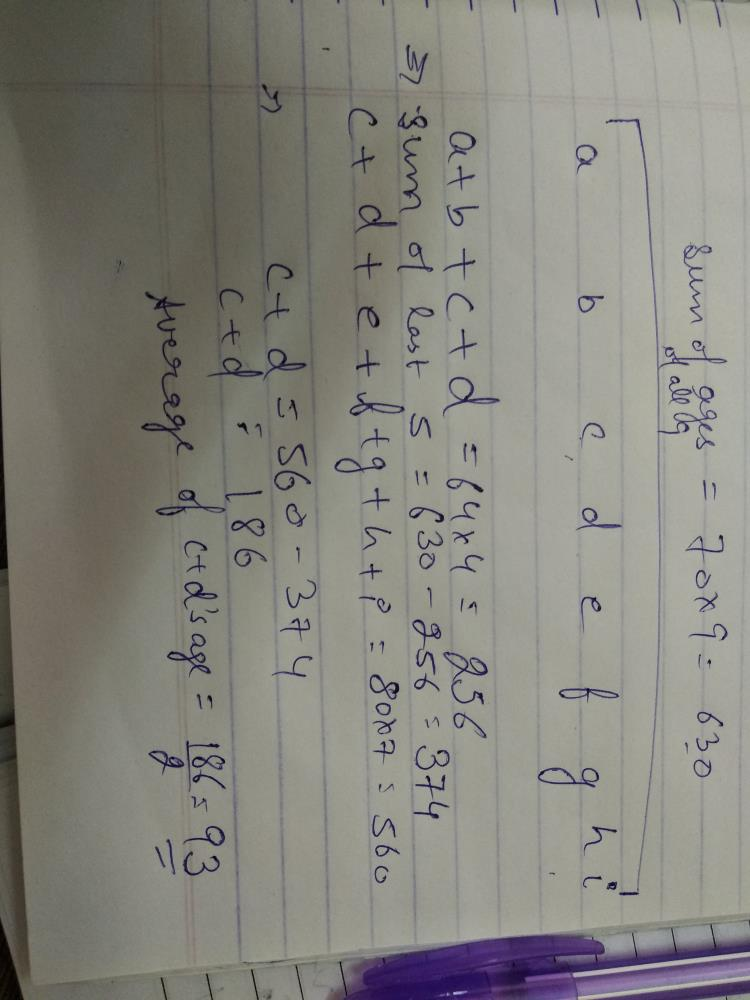Miten Shah answered Apr 23, 2020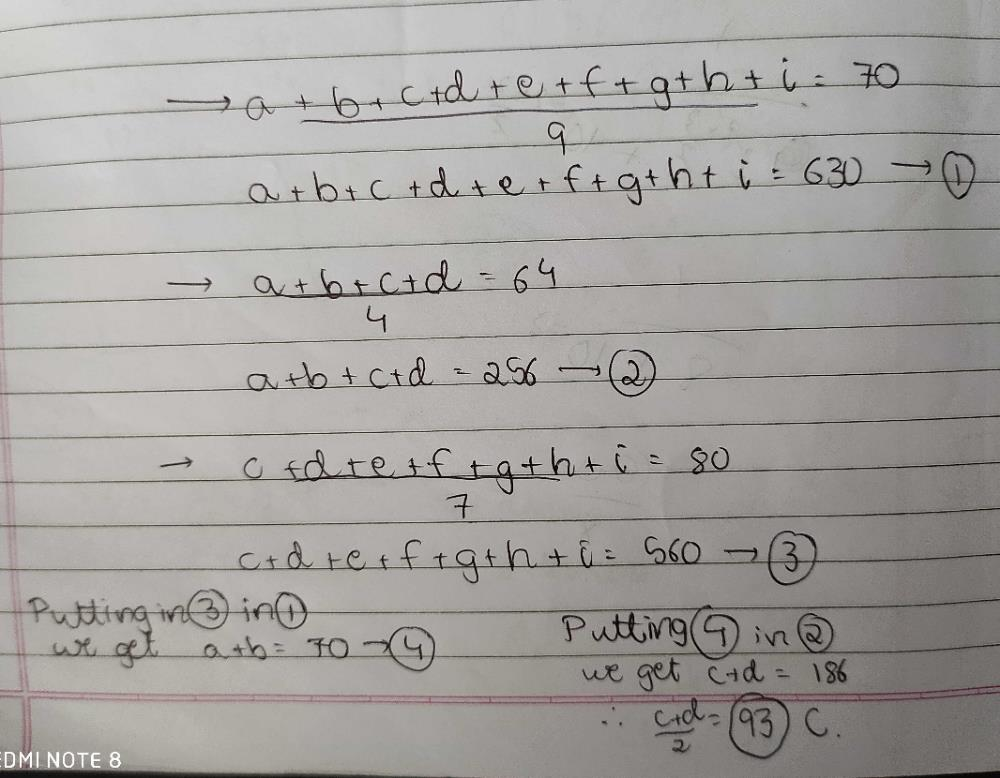Kashish Garg answered Apr 14, 2020
93

Lokesh Kumar answered Feb 28, 2020
C

Vinod Patel answered May 07, 2020
C he na shruti

Manya Mehta answered Jun 25, 2020
Simply Apply Formula of Average. BUT LOOK THE QUESTION FROM THE ANGLE OF VENN DIAGRAM IN TERMS OF SETS.

Average = Total sum of nos./ Total nos.

Here Avg. of 9 members in a row is given 70.
So Total age is 70* 9 members = 630. ( AUB )

same as above
Average of first 4 is 64.
so Total age is 64*4 members = 256. ( A )

Average of last 7 is 80.
so Total age is 80*7 members = 560. ( B )

SO ON CALCULATION WE GET
( A^B) A INTERSECTION B = (A) + (B) - (AUB)

= 256+560-630 = 186 ( TOTAL AGE OF 3RD N 4TH MEMBER IN A ROW)

THEREFORE AVERAGE AGE OF 3RD N 4TH MEMBER IN A ROW IS

186/ 2= 93

SO THE ANSWER IS OPTION C that is 93.

Ankit Sharma answered Feb 28, 2020
A

Aman Kumar answered Aug 13, 2020

Marco Polo answered Oct 16, 2020
"testing for IOS"

Manohar Mishra answered Sep 02, 2020
C pasdhiw

Pusp Kumar Vyas answered May 12, 2020
N1+n2+ ....+n9=630 --->1
n1+ ....+n4=256  --->2
n3+....+n9=560 --->3
n3+n4+(n1+....+n9)=256+560
n3+n4+630=816
n3+n4=186
(n3+n4)/2=93

Suman Devi answered Apr 03, 2020
S9/9=70
S9=630
S4/4=64
S4=256
S7/7=80
S7=560
S4+S7=256+560
=816
then,S2=816-630
=186
average of third and fourth member = 186/2=93

Roy Singh answered May 04, 2020
"9*70=630 [ 4*64=256 + 7*80=560],
(11= 816- 9 =630)

2=186
1= 93(C)"

Luvy Goel answered Jul 23, 2020
Average of first 9 number 70 so 70 ×9=630
average of first 4 number 64 so 64×4=256
average of last 7 number 80 so 80×7=560
now 560+256=630 as third and fourth number come in both first 4 and last 7
therefore 816-630 =186
as the sum of 4and5 number is 186 so the average is 186/2=93

Pranjal Srivastava answered May 30, 2020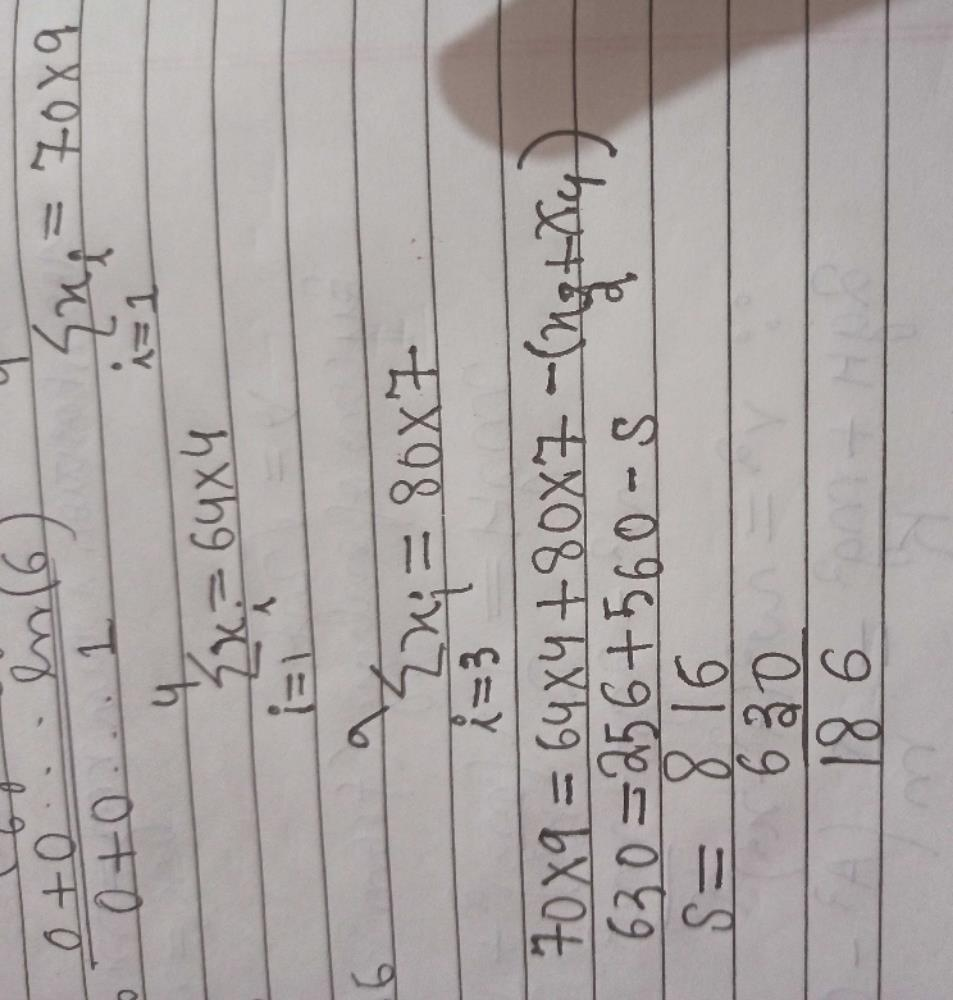Kulwinder Kaur answered Feb 20, 2020
Let the age of the members in the row be A, B, C, D, E, F, G, H and I.
∴  A + B + C + D + E + F + G + H + I = 1 0 * 9 = 630 ...(i)
Since the average age of the first four members is 64 years,
A + B + C + D = 6 4 x 4 = 256 ...(ii)
§jnce the average age of the last seven members is 80 years,
C + D + E + F + G + H + I = 80 x 7 = 560 ...(iii)
Subtracting (iii) from (i),
A + B = 70 ... (iv)
Subtracting (iv) from (ii),
C + D= 186
∴ Averageage of third and four the member =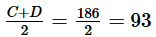Hence, option 3.

Pratishma Sidhu answered Mar 13, 2020

Tejas Thoke answered Apr 26, 2020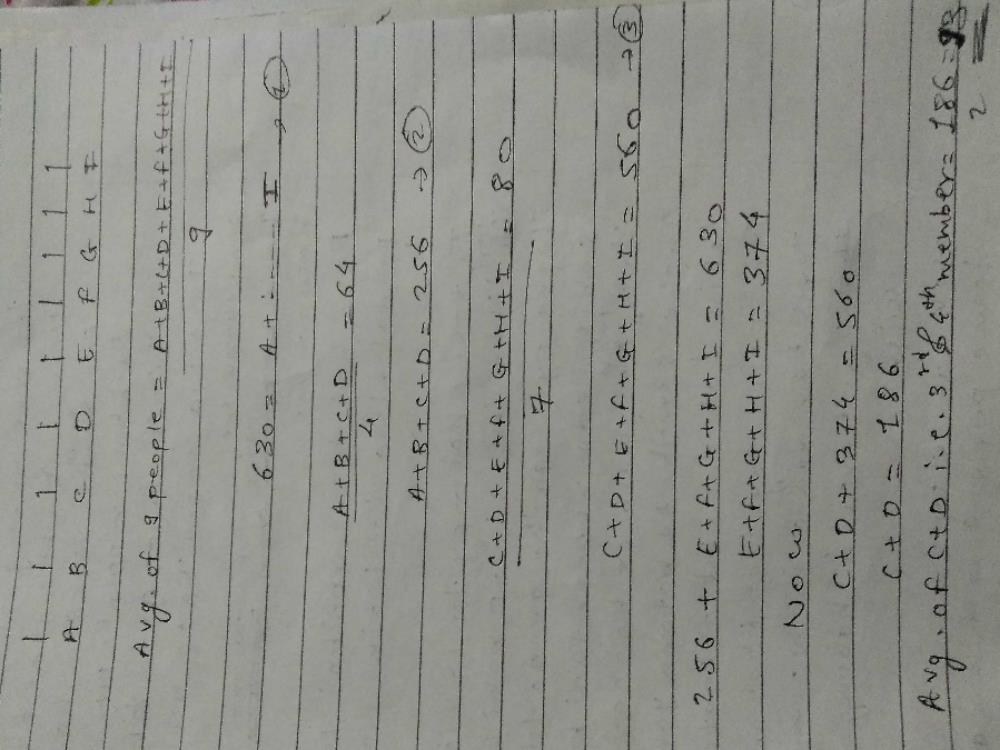Priya Tiwari answered Jun 23, 2020
70*9-80*7=70,total age of first two person.
average of 3rd or 4th = total of first 4person - total of first two person/2
= 64*4-70/2=256-70/2=186/2=93.

Mrinalini Pal answered May 20, 2020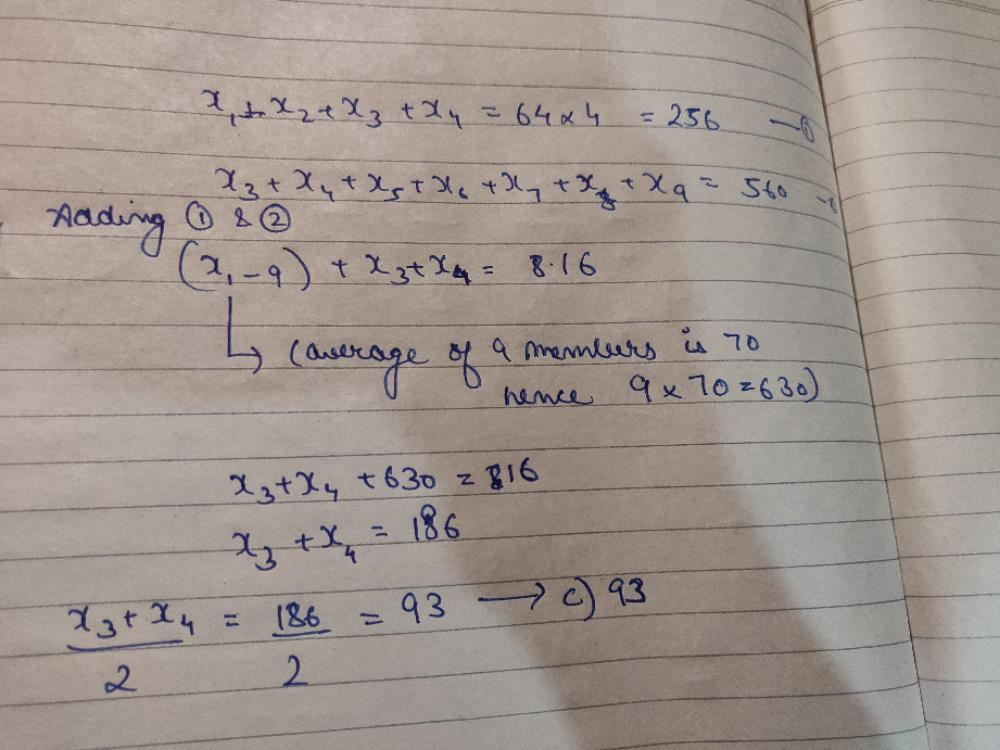Muskan Seth answered Jul 22, 2020
Average age of 9 people = 70
Therefore, total average age of 9 people = 70×9=630
average age of first four members = 64
therefore , average age of four members = 64×4= 256
average age of the last seven members = 80
therfore , average age of last seven members= 80×7 =560
AVERAGE AGE OF 9 PEOPLE- AVERAGE AGE OF LAST 7 MEMBERS = average age of first two members
therefore , 630-560=70
then .... average age of four members-average age of first two members = 256- 70 = 186 ( average age of 3rd nd 4th member )
average age of 3rd member/ 4th member = 186/2 = 93 .

Sonal Vishwakarma answered Apr 30, 2020
4*64=256
7*80=560
9*70=630
Hence, 256+560-630=186
The average age of two person would be,
186/2= 93

Shreyansh Gupta answered Sep 02, 2020

Ritu Porwal answered Sep 20, 2020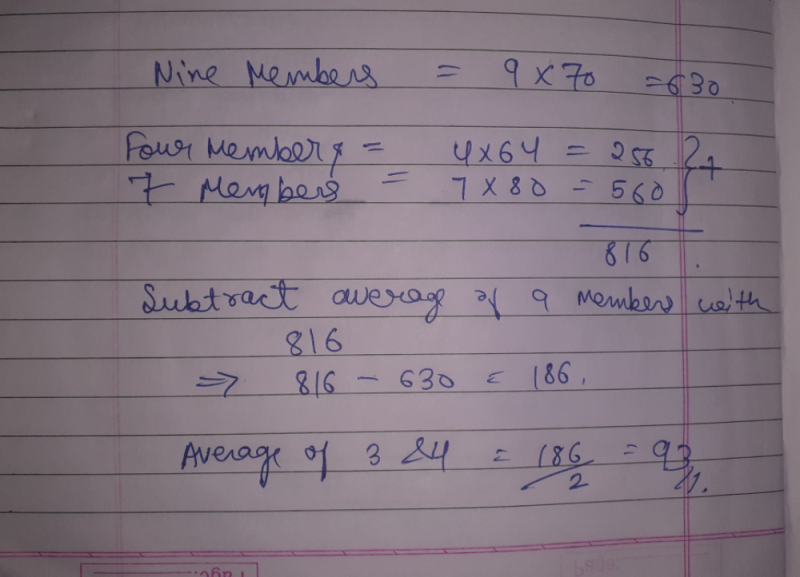Sachin Br answered Oct 06, 2020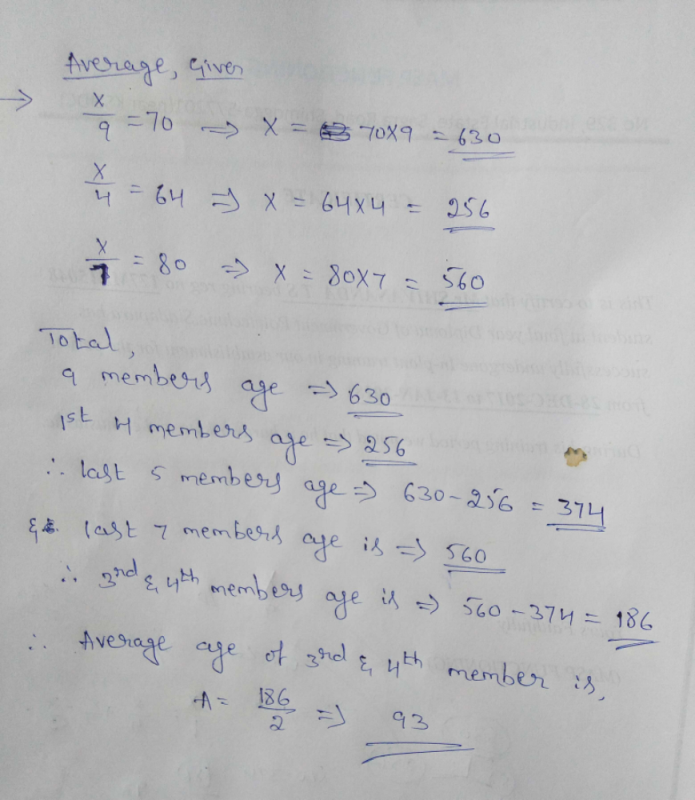Vishal Agarwal answered Mar 23, 2020
If average age of nine members of a family standing in a row = 70
Total age of nine members of the family standing in a row= 70*9=630
If average age of first four members of the family standing in a row=64
Total age of first four members of the family standing in a row=64*4=256
If average age of last seven members standing in a row = 80
Total age of last seven members standing in a row= 80*7=560
Therefore, Total age of first two members of the family standing in a row= 630-560= 70
Now, Total age of 3rd and 4th members of the family standing in a row= 256-70=186
Average age of 3rd and 4th members of the family standing in a row= 186÷2= 93

Gautami Parate answered Aug 22, 2020
Let the ages of 9 members be a,b,c,d,e,f,g,h,i resp.
According to condition,
(a+b+c+d+e+f+g+h+i)/9 = 70 ____(1)
(a+b+c+d)/4 = 64
=> a+b = 64(4)-c-d _____(2)
Also,
(c+d+e+f+g+h+i)/7 = 80
=> e+f+g+h+i = 80(7)-c-d _____(3)

put (2) and (3) in eqn (1), we get

(256-c-d+c+d+560-c-d)/9 = 70
=> 816-c-d = 70(9)
=> c+d = 816-630
=> c+d = 186

Taking Average of c and d , we get
c+d/2 = 186/2 = 93

Rajneesh 133 answered Sep 23, 2020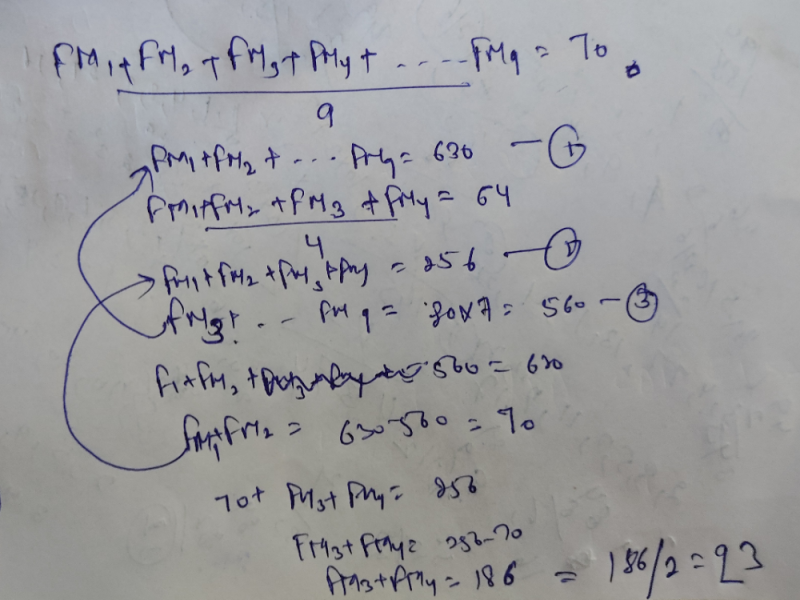Raj Kamal answered Apr 19, 2020
Average age of 3rd and 4 th member.=(sum of 3rs and 4th members' age in row) /2

to get sum of sum of 3rd and 4 th mem. age=. (sum of 4 memb. from start + sum of 7 mem. from last)- sum of 9 mem
=(256+560)-630
=816-630
186
divide by 2 we get avg.- 93

What kind of family it is ? How the average age of a family can be 80 ?

4*64=256 7*80=560 9*70=630
256+560-630=186
186/2=93

Krishna Kant Murari Raja answered May 17, 2020
9 members = Average- 70= 630
First 4 - Average- 64 = 256
Last 7- Average- 80 = 560
Therefore total = 256+560= 816
Average od 3rd & 4th = 816 - 630= 186

Monu Sharma answered Jul 06, 2020
70*9=630
and 80*7 = 560

630-560= 70. 1st and 2nd sum

so 64*4= 256

3rd + 4th sum = 256-70= 186

avg= 186/2= 93

Kalaga Akshay answered Jul 08, 2020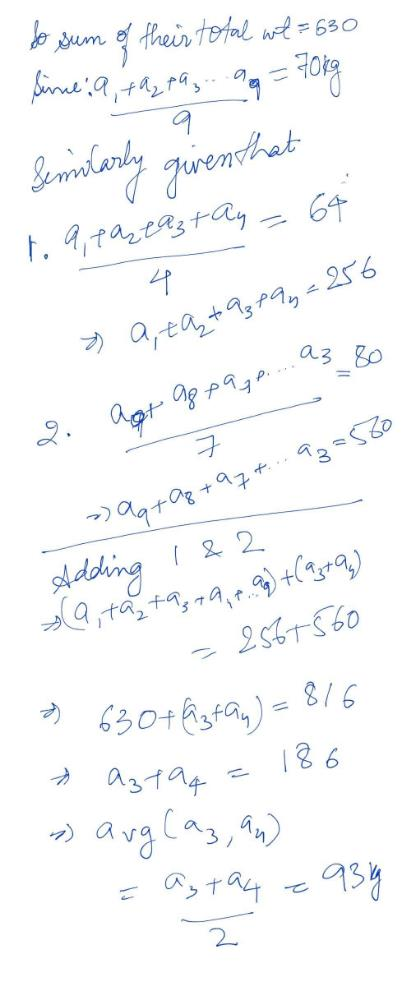Dipak Potale answered Jul 31, 2020
D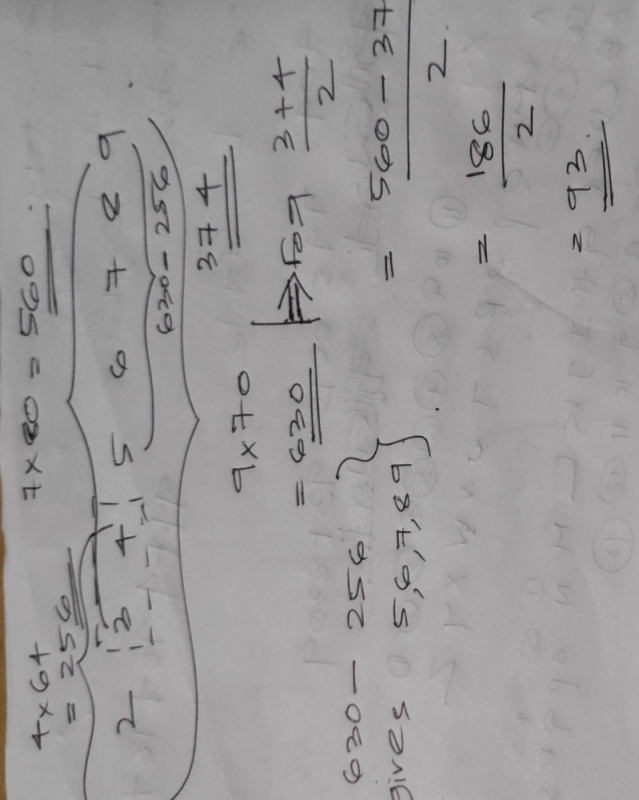Ann Mary answered Oct 02, 2020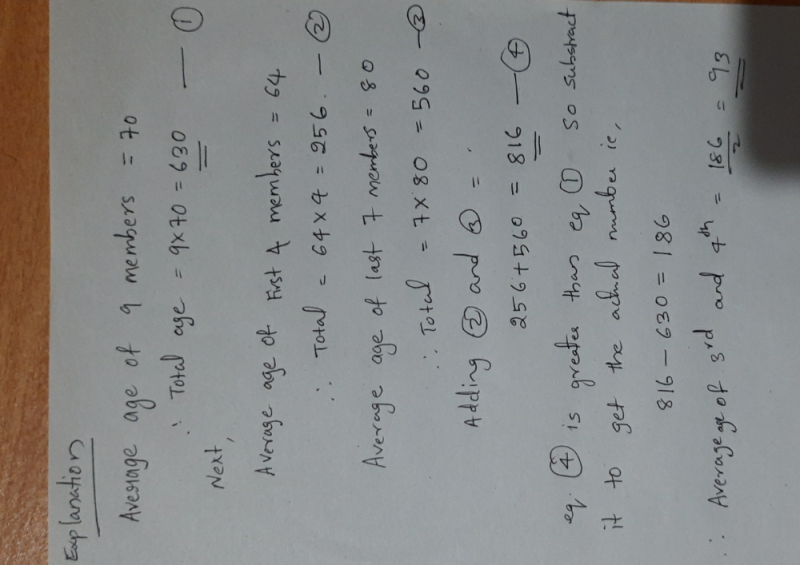Abhishek Kumar answered Apr 23, 2020
First sum of the ages of 9 people= 70×9=630
second Sum of the ages of first 4 people =64×4=256
Third sum of the ages of last 7 people =80×7=560
subtract the sum of the ages of 7 people from ages of 9 people.= 630- 560=70. this is the value of first 2 people
And substitute this value in the sum of the ages of first four peopl
Let the people are t1, t2, t3, t9
t1+.....+t9=630
t3+...+t9=560
subtract this t1+t2= 70
and
t1+t2+t3+t4=256
70+t3+t4=256
t3+t4=186
then Avg. of t3 and t4 is 186/2 =93
the ansr is 93

Abhishek Kumar answered Apr 22, 2020
Total marks of first four=64*4=256
Total marks of last 7=80*7=560
By Adding both you will get 256+560=816
Now the total marks of all 9 students =70*9=630
So there are difference of 186 and we now that third and fourth counts double so third and fourth both are equal to 816-630=186 .
It is the total value of both 3rd and 4th. So obviously the average for third and fourth is 186/2=93.

Yukti Sharma answered May 14, 2020

Vivek Singh answered May 08, 2020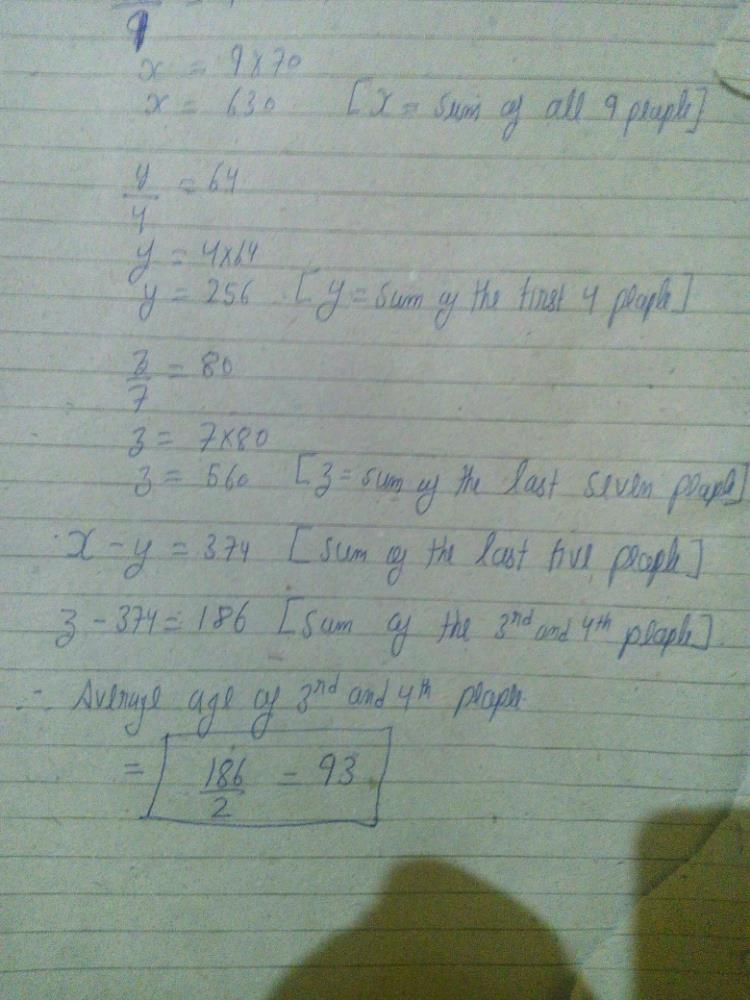Nitish Mishra answered Sep 15, 2020

Mayur Nikam answered Sep 24, 2020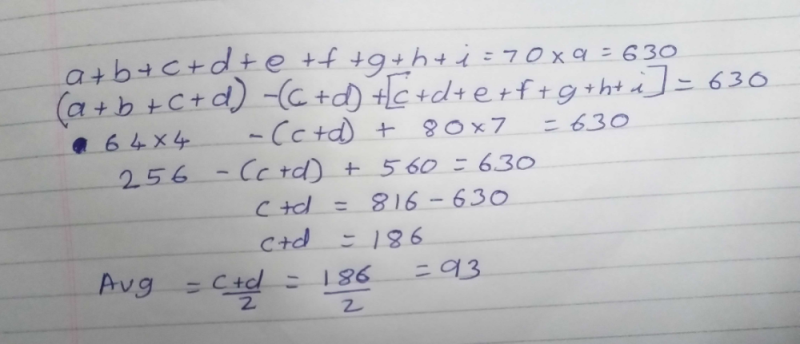Swetha Viriyala answered Jul 11, 2020
Assume the persons as A1,A2,A3.....A9
Given average of A1 till A9 is 70. So sum of all is 70*9 = 630 (1)

Given average of first 4 i.e., A1 to A4 is 64. So sum of A1 to A4 is 64*4 = 256 (2)

Given average of last 7 i.e., A3 to A9 is 80. So sum of A3 to A9 is 80*7 = +560 (3)

We can see that A1 to A9 + A3 + A4 will be the sum = 256+560 = 816 (4)

(4)-(1) = A3 + A4 (since (1) = sum of A1 to A9) = 816 - 630 = 186

Now, average of A3 & A4 = 186/2 = 93 -> Ans

Mamata Das answered Jul 10, 2020
C. 93

Sneha Banerjee answered Jul 06, 2020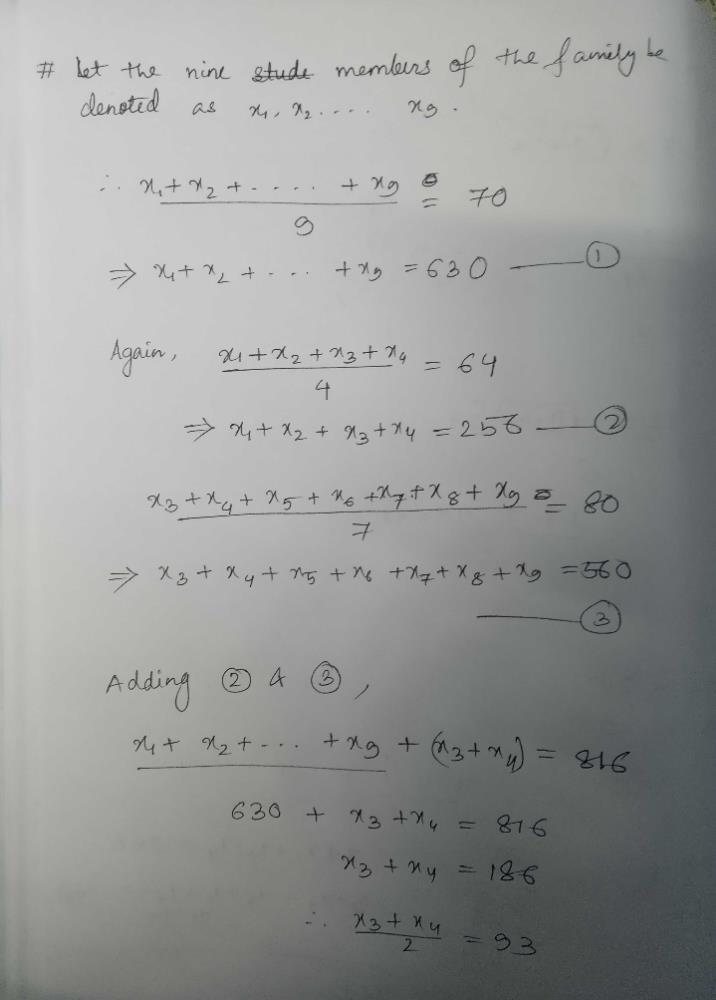Garima Pal answered May 13, 2020
Let the age of nine people be a,b,c,d,e,f,g,h and i
Subtract the sum of last 7 no.s (80*7) from the sum of 9 no.s (70*9) then you will get the sum of a+b =70 .
Now subtract the sum of a+b from the sum of a+b+c+d(64*4) .
you will get c+d =186
now the average of third and fourth that is c+d will be 186/2=93 ( option c)

Rashi Chandra answered Aug 07, 2020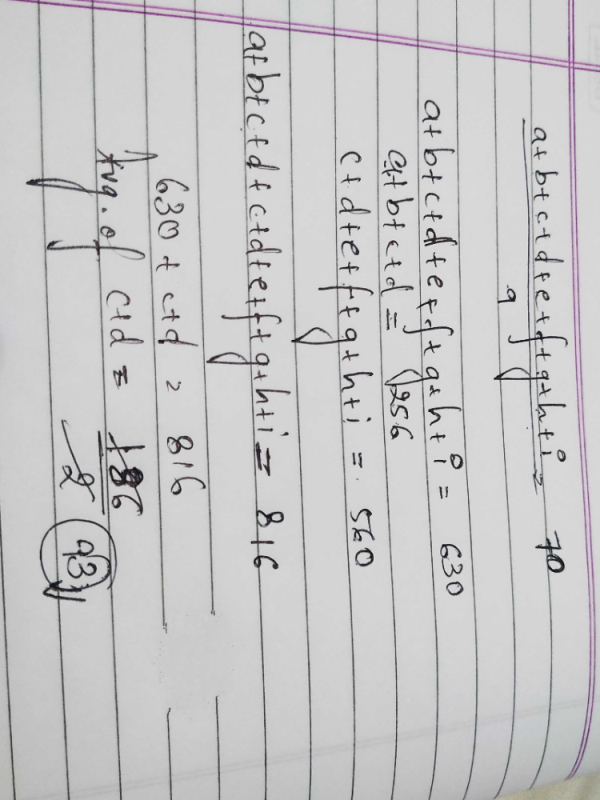Khushi Popat answered Aug 06, 2020
Total age of members=average × no. of members
i.e

70×9 =630.

given that: the average age of 1st four member is 64

64×4 =256. (total age of member 1 to 4)

therefore the age of last 5 members will be

630-256= 374. (total age of member 5 to 9)

given that: the average age of the last seven members i.e member 3 to 9 is 80

80×7=560. (total age of member 3 to 9)

to get the total age of 3rd and 4th member

560-374=186

average = 186/2 =93. option c

Sum of age of 9 members=630
sum of age of first 4 members= 256 @1
sum of age of last seven members =560 @2

560+256-630 gives you 186 sum of age of 3&4 member
average of 3&4 member will be
186÷2 =93

Tarun Sharma answered Jul 13, 2020
Average age of 9 members is 70 then total of there age would be 630.
average age of first four members is 64 then total of there age would be 256
average age of last seven members is 80 so total of there age would be 560
total of age of first two members = total age of 9 member - total age of last seven members = 630-540=70
than total age of 3rd +4th member of row = 256-70=186
than average age would be 186/2= 93 answer c is correct.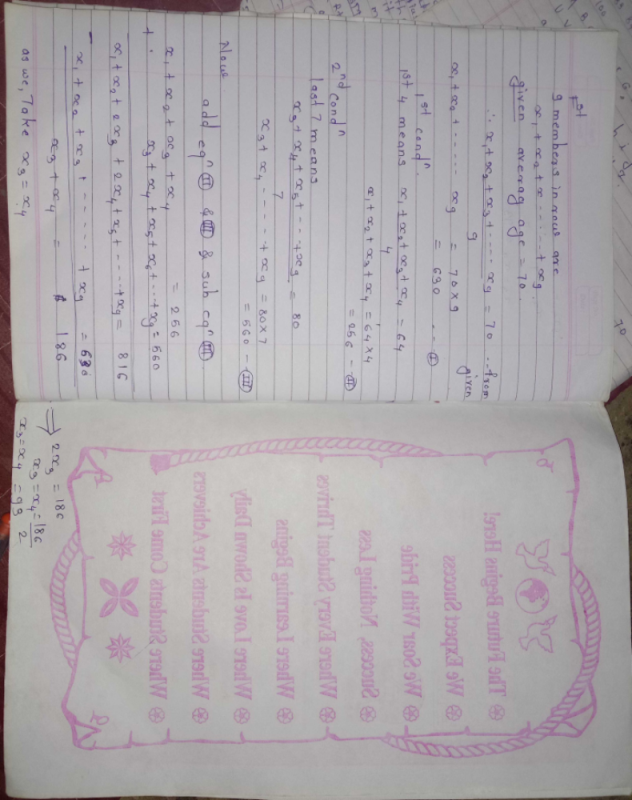Divyansh Chandak answered Apr 19, 2020
Total age=630
total age of first 4 - 256
total age of last 7 - 560
required average -- 560+256-630/2----93

Babita Banik answered Jul 27, 2020

Chouda Sowsheel answered Jun 11, 2020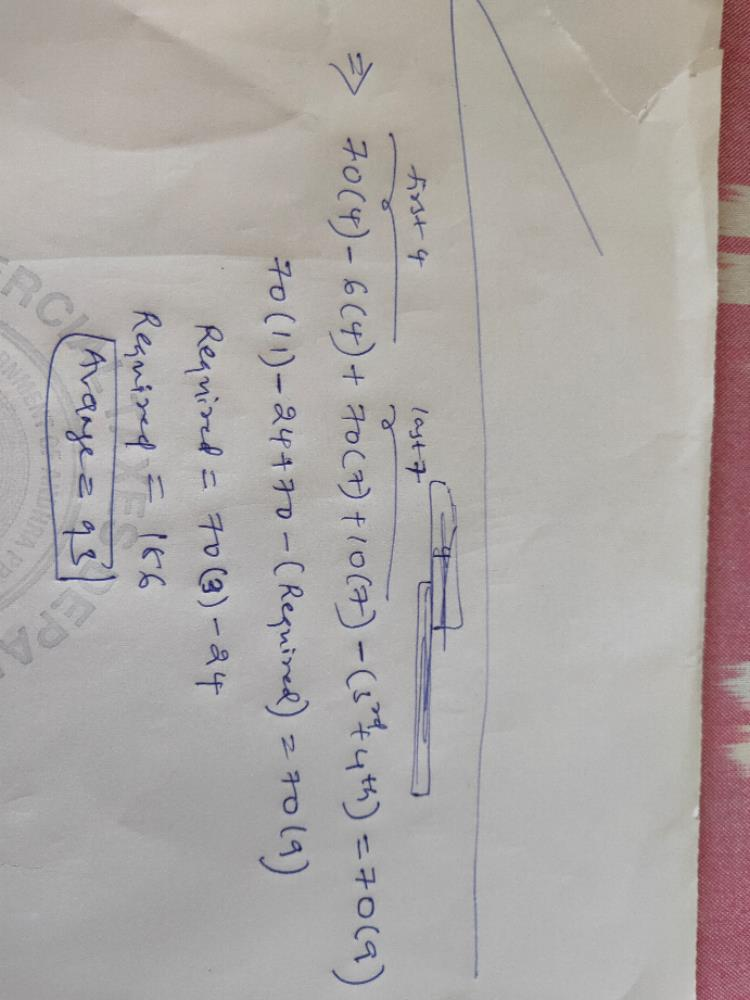Sayonie Chakrabarty answered May 05, 2020
Ans is 93

Sachin Kamble answered Aug 03, 2020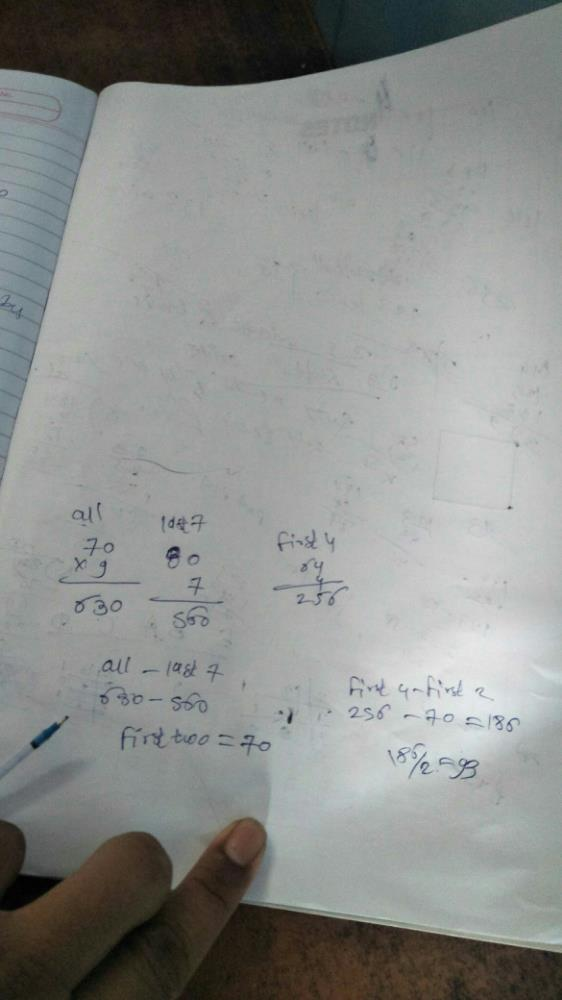Ankit Verma answered Aug 13, 2020
Sum of 9 = 70*9=630
sum of first 4 = 64*4 = 256
sum of last 7 = 80*7 = 560
sum of first 4 and last 7 = 256+ 560 = 816
sum of 3rd and 4th number= 816 - 630 = 186
average of 3rd and 4th number = 186/2 = 93

Raksha Saxena answered Sep 18, 2020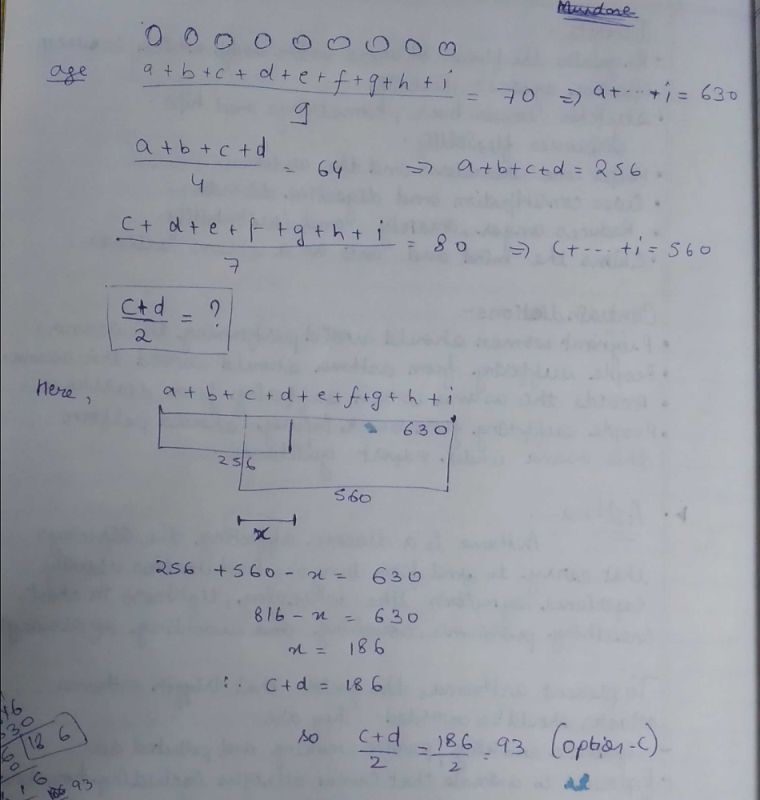Anshul Sancheti answered 4 weeks ago
Let 9 members be A,B,C,D,E,F,G,H,I
A+B+C+D+E+F+G+H+I = 9*70 => 630 (given) (i)
A+B+C+D = 4*64 => 256 (given). (ii)
C+D+E+F+G+H+I = 7*80 => 560 (given). (iii)
A+B+C+D+C+D+E+F+G+H+I = 816
Now Subtract it from (i)
(A+B+C+D+C+D+E+F+G+H+I) - (A+B+C+D+E+F+G+H+I)= 816-630
C+D = 186
Average would be 186/2 = 93 Years

Anumol Thankappan answered 6 days ago
9 members x 70yrs(avg) = 630 --- (1)

4 members x 64 yrs(avg) = 256 --- (2)

7 members x 80 yrs(avg) = 560 --- (3)
.
(2) + (3) = 11 members with sum of age as 816 --- (4)

(4) - (1) = 2 members ie; sum of ages of 3rd and 4th person= 186. Hence, average= 186/2= 93 years. Answer is 93.

Veera Reddy Konabhai answered Feb 10, 2020
Consider first two members as set A, third person as B, fourth person as C and remaining five membes as set D.
given (A+B+C+D)/9 = 70 => A+B+C+D = 630
and (A+B+C)/4 = 64 => A+B+C = 256 ----> smt1
and (B+C+D)/7 = 80 => B+C+D = 560 ----->smt2
now add smt1 and smt2 that gives us A+B+C+B+C+D = 816 and given A+B+C+D is 630 subitting in previous equation we get B+C+630 = 816 and solving gives us B+C = 186. now we want avg of B,C that is (B+C)/2 = 186/2 = 93

Shikhar Gupta answered Jun 21, 2020
C) 93

Anshi Kaa answered Jun 20, 2020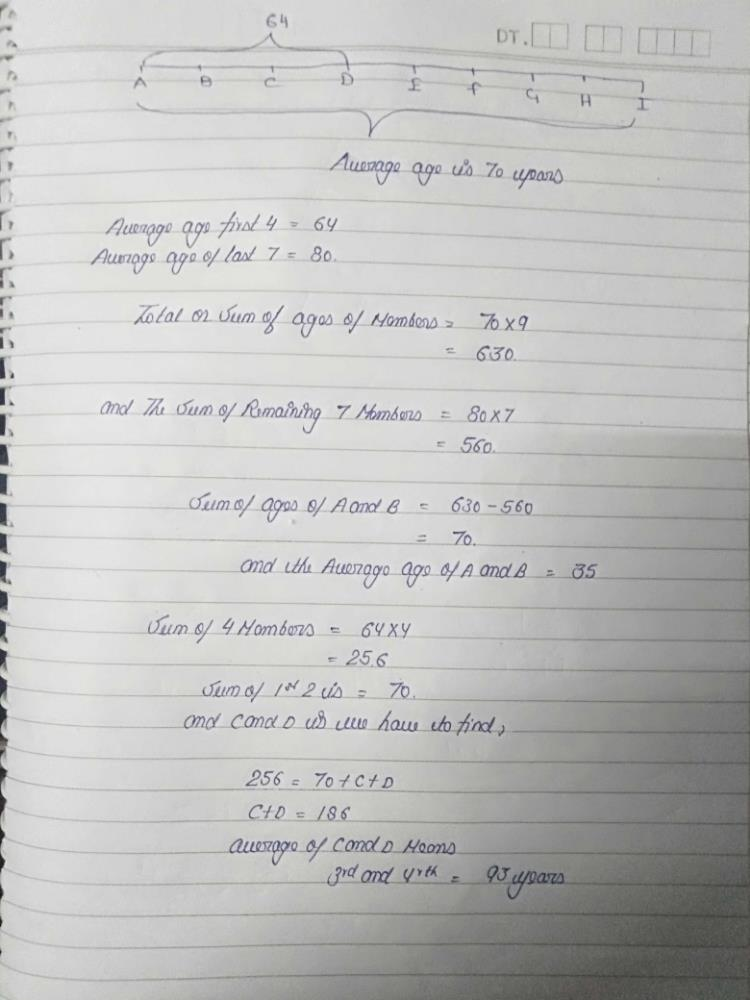Shubham Chavan answered Jun 23, 2020
E

Nupur Goyal answered Sep 18, 2020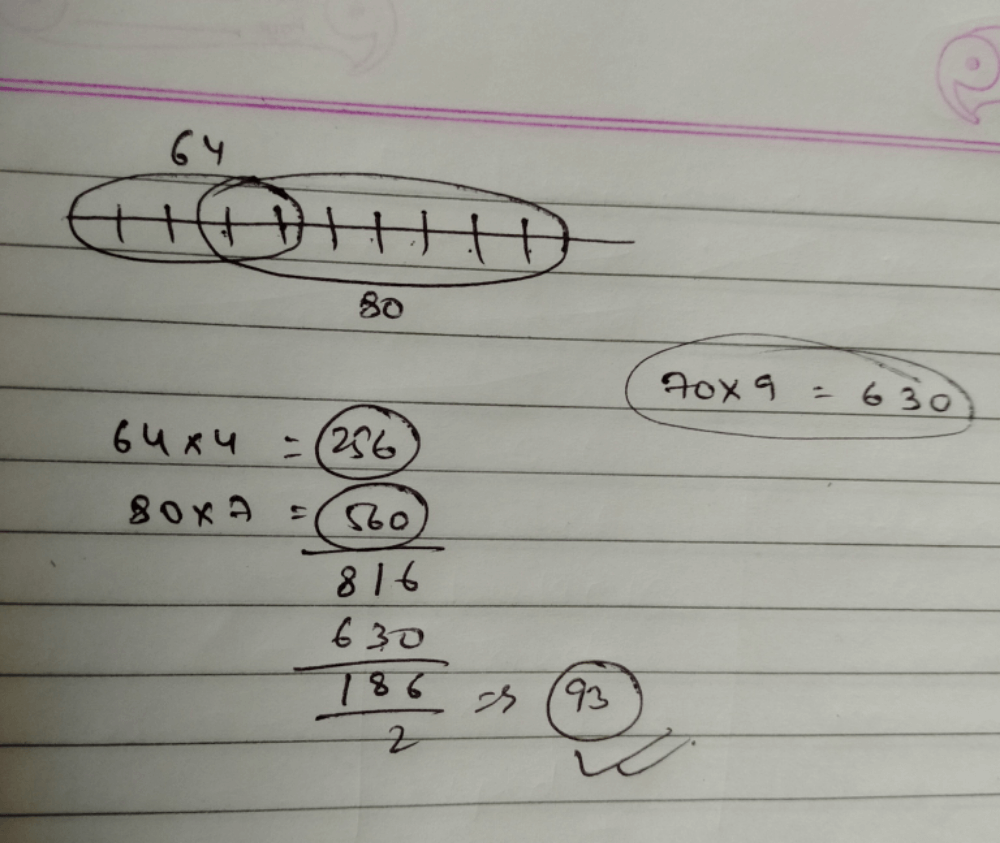9×70 = 630

64×4= 256
80×7 = 560

Therefore sum of third and fourth age = (256+560) - 630

= 186/ 2 = 93

Rishita Roy answered Apr 27, 2020
Let the 9 members be A, B, C, D, E, F, G, H, I. Now the number of member's ages divided by 9 is equal to 70. So the sum of the ages will be 70*9=630. Now, average age of first 4 members is equal to 64. Therefore the sum of the age of the first 4 members is 64*4=256. Again, average age of the last 7 members is 80. So the sum of the age of the members is 80*7=560. Now put the age value of the first 4 members in the sum of all the 9 members , ie, A+B+C+D+E+F+G+H+I=630.
or, 256+E+F+G+H+I=630
or, E+F+G+H+I=630-256=374.
Now, Put the above found age value in the sum of the last 7 members, ie,
C+D+E+F+G+H+I=560
or, C+D+374=560
or, C+D=560-374=186
Average of C+D=186/2=93.
So the average age of the third and fourth member is 93.

Masnoon Alam answered Jun 09, 2020
68

Miss Rai answered Jul 21, 2020
We can use the de Morgan's law in this.
as we know
n(AUB)=n(A)+n(B)-n(A^B)
so we have
A=set of first 4 members
B=set of last seven members
AUB=Set of all 9 members
note that when we say set we are talking about the sum
I.e. n(A)=64×4
n(B)=80×7
n(AUB)=70×9
n(A^B)=186
3rd+4th)=186÷2
=93

Suyog Gandhi answered Jul 14, 2020
Hence ans is C.

Navneet Mishra answered Jun 29, 2020
Let the members are :-
a1, a2, a3, a4, a5, a6, a7, a8, a9
Avg9 :- (a1+a2+a3+a4+a5+a6+a7+a8+a9)/9 =70
a1+a2+a3+a4+a5+a6+a7+a8+a9 = 630 (1)

Avg4:- (a1+a2+a3+a4)/4 = 64
a1+a2+a3+a4 = 256 (2)
From eq(1) - (2)
a5+a6+a7+a8+a9 =374 (3)

Avg_last7:- (a3+a4+a5+a6+a7+a8+a9)/7 = 80
a3+a4+a5+a6+a7+a8+a9 = 560 (4)
From eq(4) - eq(3)
a3+a4 = 186
(a3+a4)/2 =186/2
Avg of 3 and 4 members :- (a3+a4)/2 = 93
Option(c)

Akshay Jedhe answered Jul 06, 2020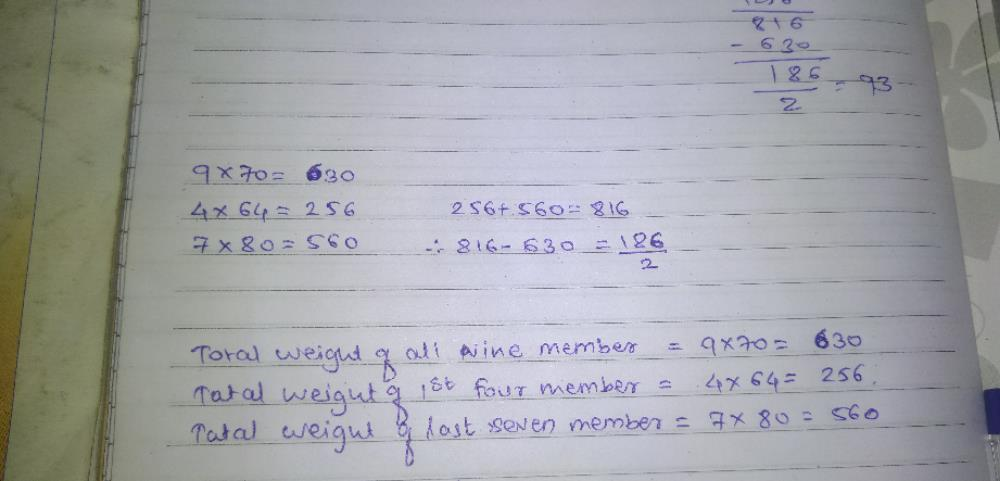Sonu Kumar answered Jun 30, 2020

Fathima Sahla answered 2 days ago
The average age of 9 members is 70
So s1+s2+to s9=70=70×9=630
as the average age of last 7 member is 80
80×7=560 now
630-560=70
which is the combined age of s1and s2 as it is given average of first 4 members
is 64 So the combined age is 256
from which 256-70=186
which is combined age of s3 and s4 So the average is 186÷2=93.